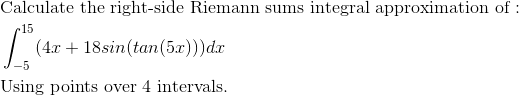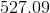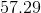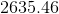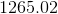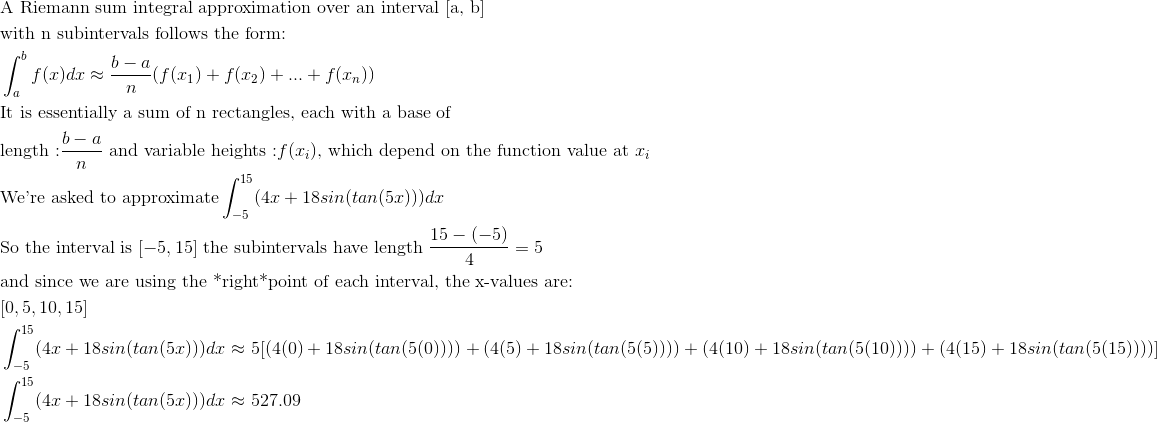# AP Calculus BC : Riemann Sum: Right Evaluation

## Example Questions

← Previous 1

### Example Question #4 : Riemann Sums

Given a function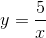, find the Right Riemann Sum of the function on the interval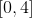divided into four sub-intervals.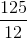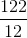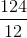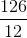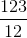Explanation:

In order to find the Riemann Sum of a given function, we need to approximate the area under the line or curve resulting from the function using rectangles spaced along equal sub-intervals of a given interval. Since we have an intervaldivided intosub-intervals, we'll be using rectangles with vertices at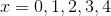.

To approximate the area under the curve, we need to find the areas of each rectangle in the sub-intervals. We already know the width or base of each rectangle is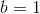because the rectangles are spacedunit apart. Since we're looking for the Right Riemann Sum of, we want to find the heightsof each rectangle by taking the values of each rightmost function value on each sub-interval, as follows: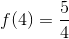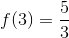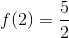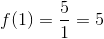Putting it all together, the Right Riemann Sum is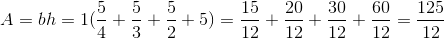.

### Example Question #1 : Riemann Sum: Right Evaluation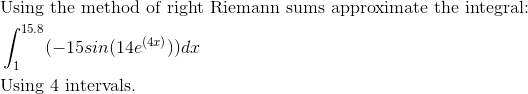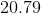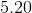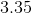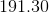Explanation: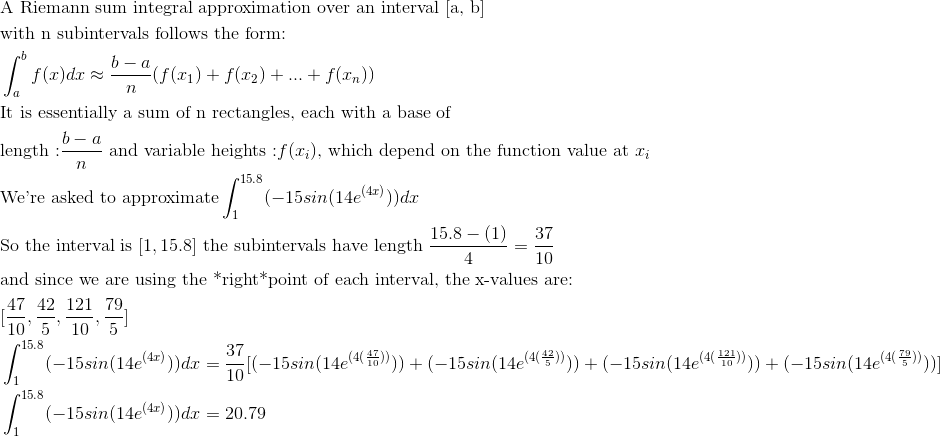### Example Question #2 : Riemann Sum: Right Evaluation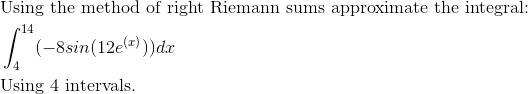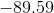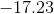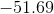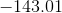Explanation: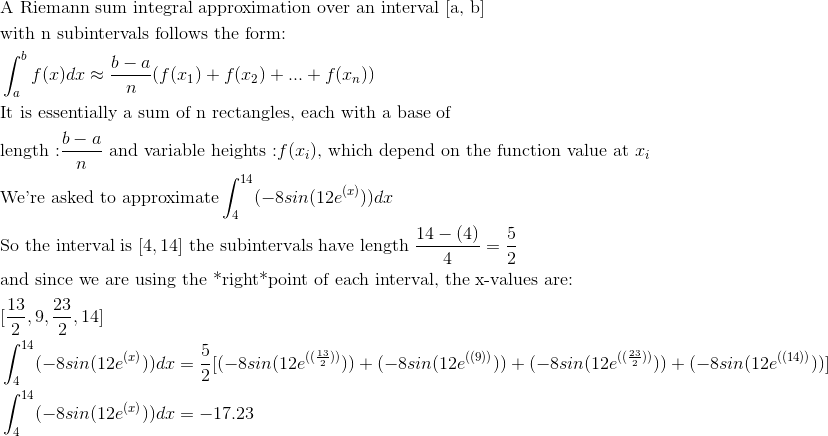### Example Question #3 : Riemann Sum: Right Evaluation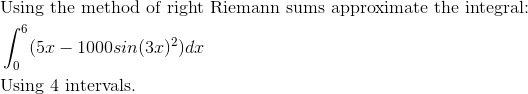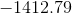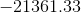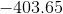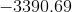Explanation: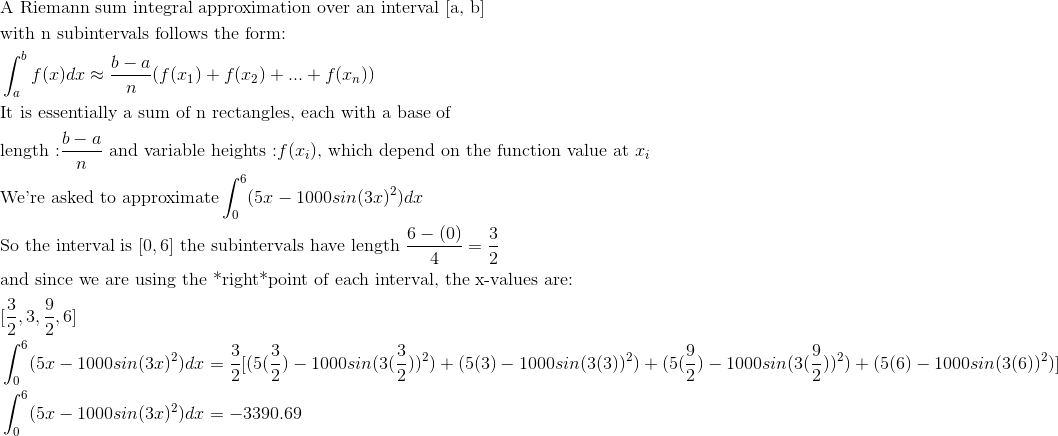### Example Question #4 : Riemann Sum: Right Evaluation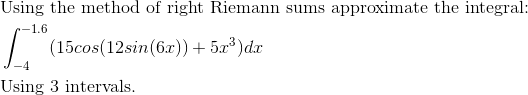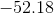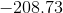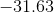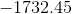Explanation: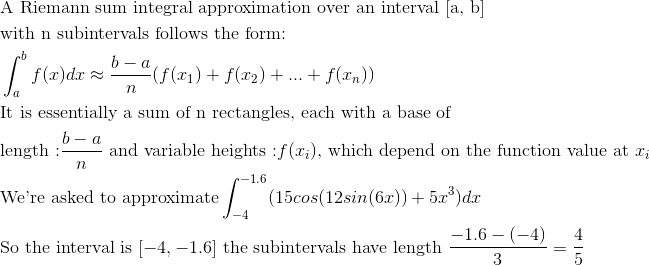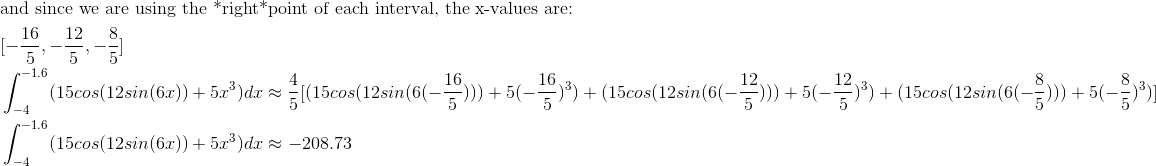### Example Question #5 : Riemann Sum: Right Evaluation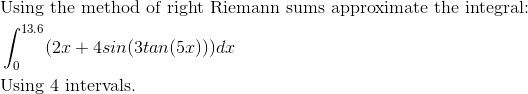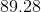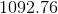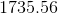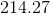Explanation: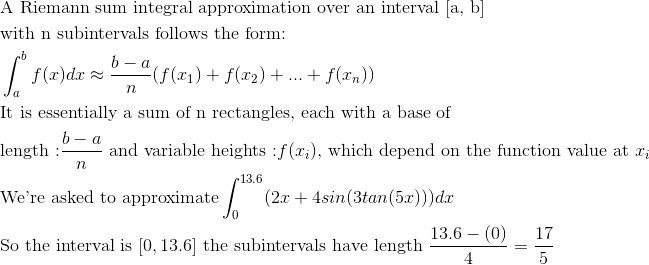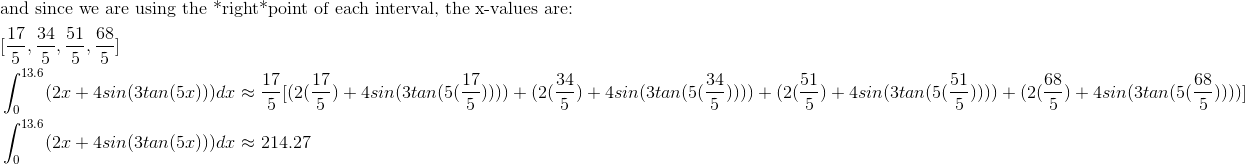### Example Question #6 : Riemann Sum: Right Evaluation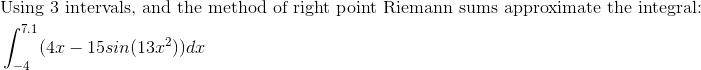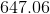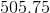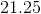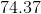Explanation: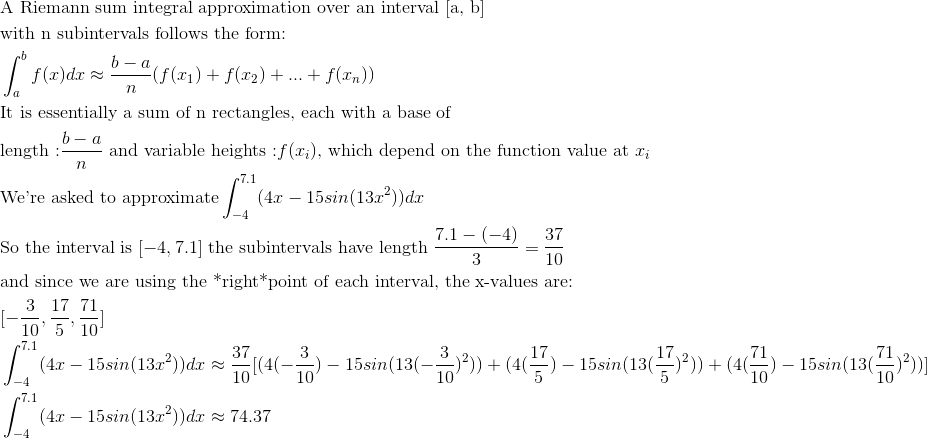### Example Question #7 : Riemann Sum: Right Evaluation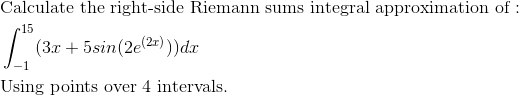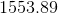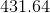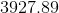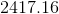Explanation: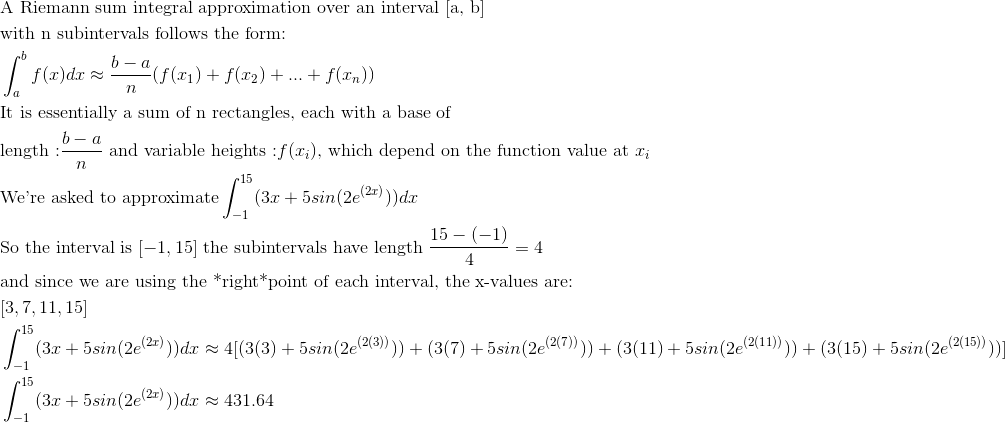### Example Question #8 : Riemann Sum: Right Evaluation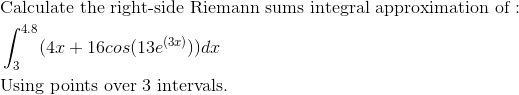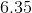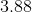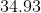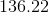Explanation: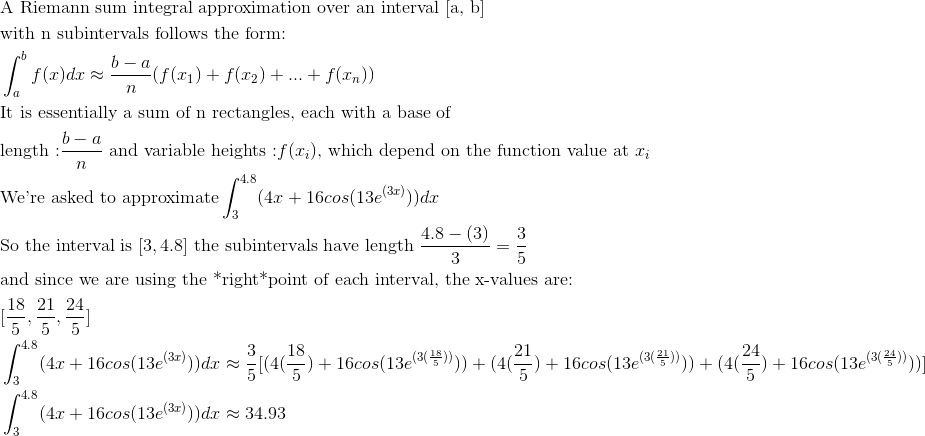### Example Question #9 : Riemann Sum: Right Evaluation